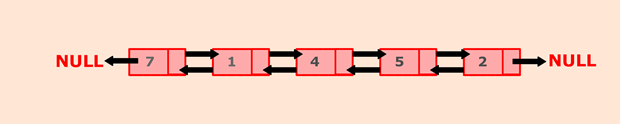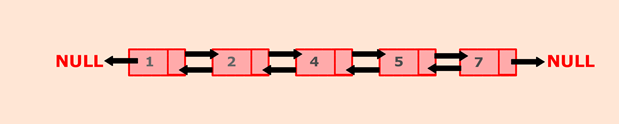Q:

# Java program to sort the elements of the doubly linked list

belongs to collection: Java Doubly Linked List Programs

In this program, we will create a doubly linked list and sort nodes of the list in ascending order.

Original List:Sorted List:To accomplish this, we maintain two pointers: current and index. Initially, current point to head node and index will point to node next to current. Traverse through the list till current points to null, by comparing current's data with index's data. If the current's data is greater than the index's data, then swap data between them. In above example, current will initially point to 7, and the index will point to 1. Since, 7 is greater than 1, swap the data. Continue this process till the entire list is sorted in ascending order.

### Algorithm

• Define a Node class which represents a node in the list. It will have three properties: data, previous which will point to the previous node and next which will point to the next node.
• Define another class for creating a doubly linked list, and it has two nodes: head and tail. Initially, head and tail will point to null.
• It first checks whether the head is null, then it will insert the node as the head.
• Both head and tail will point to a newly added node.
• Head's previous pointer will point to null and tail's next pointer will point to null.
• If the head is not null, the new node will be inserted at the end of the list such that new node's previous pointer will point to tail.
• The new node will become the new tail. Tail's next pointer will point to null.

a. sortList() will sort nodes of the list in ascending order.

• Define a node current which will point to head.
• Define another node index which will point to node next to current.
• Compare data of current and index node. If the current's data is greater than the index's data, then swap the data between them.
• Current will point to current.next and index will point to index.next.
• Continue this process till the entire list is sorted.

a. display() will show all the nodes present in the list.

• Define a new node 'current' that will point to the head.
• Print current.data till current points to null.
• Current will point to the next node in the list in each iteration.

### Program:

``````public class SortList {

//Represent a node of the doubly linked list

class Node{
int data;
Node previous;
Node next;

public Node(int data) {
this.data = data;
}
}

//Create a new node
Node newNode = new Node(data);

//If list is empty
//Both head and tail will point to newNode
//head's previous will point to null
//tail's next will point to null, as it is the last node of the list
tail.next = null;
}
else {
//newNode will be added after tail such that tail's next will point to newNode
tail.next = newNode;
//newNode's previous will point to tail
newNode.previous = tail;
//newNode will become new tail
tail = newNode;
//As it is last node, tail's next will point to null
tail.next = null;
}
}

//sortList() will sort the given list in ascending order
public void sortList() {
Node current = null, index = null;
int temp;
//Check whether list is empty
return;
}
else {
for(current = head; current.next != null; current = current.next) {
//Index will point to node next to current
for(index = current.next; index != null; index = index.next) {
//If current's data is greater than index's data, swap the data of current and index
if(current.data > index.data) {
temp = current.data;
current.data = index.data;
index.data = temp;
}
}
}
}
}

//display() will print out the nodes of the list
public void display() {
//Node current will point to head
System.out.println("List is empty");
return;
}
while(current != null) {
//Prints each node by incrementing the pointer.

System.out.print(current.data + " ");
current = current.next;
}
System.out.println();
}

public static void main(String[] args) {

SortList dList = new SortList();

//Displaying original list
System.out.println("Original list: ");
dList.display();

//Sorting list
dList.sortList();

//Displaying sorted list
System.out.println("Sorted list: ");
dList.display();
}
}  ``````

Output:

```Original list:
7 1 4 5 2
Sorted list:
1 2 4 5 7 ```